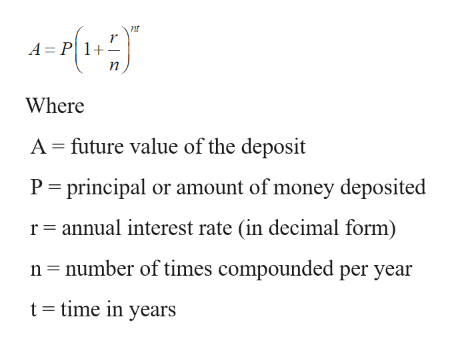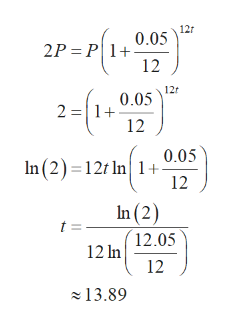# Use the compound interest formula to determine how long it will take for a sum of money to double if it is invested at a rate of 5% per year compounded monthly. (Round your answer to two decimal places.)

Question
1 views

Use the compound interest formula to determine how long it will take for a sum of money to double if it is invested at a rate of 5% per year compounded monthly. (Round your answer to two decimal places.)

check_circle

Step 1

Formula for compound interest:help_outlineImage TranscriptioncloseA = P 1+ Where A = future value of the deposit P = principal or amount of money deposited r= annual interest rate (in decimal form) n= number of times compounded per year t= time in years fullscreen
Step 2

We have A = 2P, r = 0.05, n = 12

Substitute the abov...help_outlineImage Transcriptionclose12r 0.05 2P = P|1+ 2-(1- 12r 0.05 2 =|1+ 12 0.05 In (2)=12t In 1+ 12 In (2) t = 12.05 12 In 12 - 13.89 fullscreen

### Want to see the full answer?

See Solution

#### Want to see this answer and more?

Solutions are written by subject experts who are available 24/7. Questions are typically answered within 1 hour.*

See Solution
*Response times may vary by subject and question.
Tagged in

### Applications of Mathematics